#### STLC: Controllability that handles obstacles

The controllability concept discussed so far has no concern for how far the trajectory travels inbefore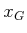is reached. This issue becomes particularly important for underactuated systems and planning among obstacles. These concerns motivate a natural question: Is there a form of controllability that is naturally suited for obstacles? It should declare that if a state is reachable from another in the absence of differential constraints, then it is also reachable with the given system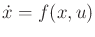. This can be expressed using time-limited reachable sets. Let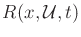denote the set of all states reachable in time less than or equal to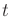, starting from. A systemis called small-time locally controllable (STLC) from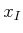if there exists some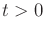such that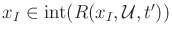for all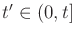(here,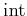denotes the interior of a set, as defined in Section 4.1.1). If the systemis STLC from every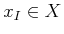, then the whole system is said to be STLC.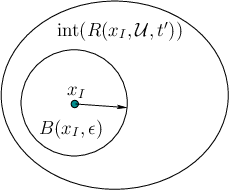Consider using this definition to answer the question above. Since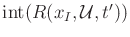is an open set, there must exist some small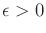for which the open ball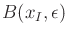is a strict subset of. See Figure 15.2. Any point on the boundary ofcan be reached, which means that a step of size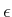can be taken in any direction, even though differential constraints exist. With obstacles, however, we have to be careful that the trajectory fromto the surface ofdoes not wander too far away.

Suppose that there is an obstacle region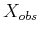, and a violation-free state trajectory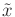is given that terminates inat time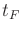and does not necessarily satisfy a given system. If the system is STLC, then it is always possible to find another trajectory, based on, that satisfies the differential constraints. Apply the plan-and-transform method of Section 14.6.2. Suppose that intervals for potential replacement are chosen using binary recursive subdivision. Also suppose that an LPM exists that computes that shortest trajectory between any pair of states; this trajectory ignores obstacles but respects the differential constraints. Initially,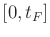is replaced by a trajectory from the LPM, and if it is not violation-free, thenis subdivided into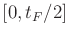and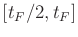, and replacement is attempted on the smaller intervals. This idea can be applied recursively until eventually the segments are small enough that they must be violation-free.

This final claim is implied by the STLC property. No matter how small the intervals become, there must exist a replacement trajectory. If an interval is large, then there may be sufficient time to wander far from the original trajectory. However, as the time interval decreases, there is not enough time to deviate far from the original trajectory. (This discussion assumes mild conditions on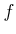, such as being Lipschitz.) Suppose that the trajectory is protected by a collision-free tube of radius. Thus, all points along the trajectory are at leastfrom the boundary of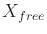. The time intervals can be chosen small enough to ensure that the trajectory deviations are less thanfrom the original trajectory. Therefore, STLC is a very important property for a system to possess for planning in the presence of obstacles. Section 15.4 covers some mathematical tools for determining whether a nonlinear system is STLC.

A concept closely related to controllability is accessibility, which is only concerned with the dimension of the reachable set. Letbe the dimension of. If there exists somefor which the dimension of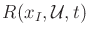is, then the system is called accessible from. Alternatively, this may be expressed as requiring that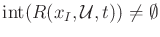.

Example 15..3 (Accessibility)   Recall the system from Section 13.1.3 in which the state is trapped on a circle. In this case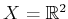, and the state transition equation was specified by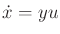and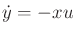. This system is not accessible because the reachable sets have dimension one.A small-time version of accessibility can also be defined by requiring that there exists somesuch that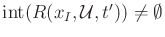for all. Accessibility is particularly important for systems with drift.

Steven M LaValle 2020-08-14